Question

Use the data below, for 298.15 K, to calculate the thermodynamic equilibrium constant, kp, at 839...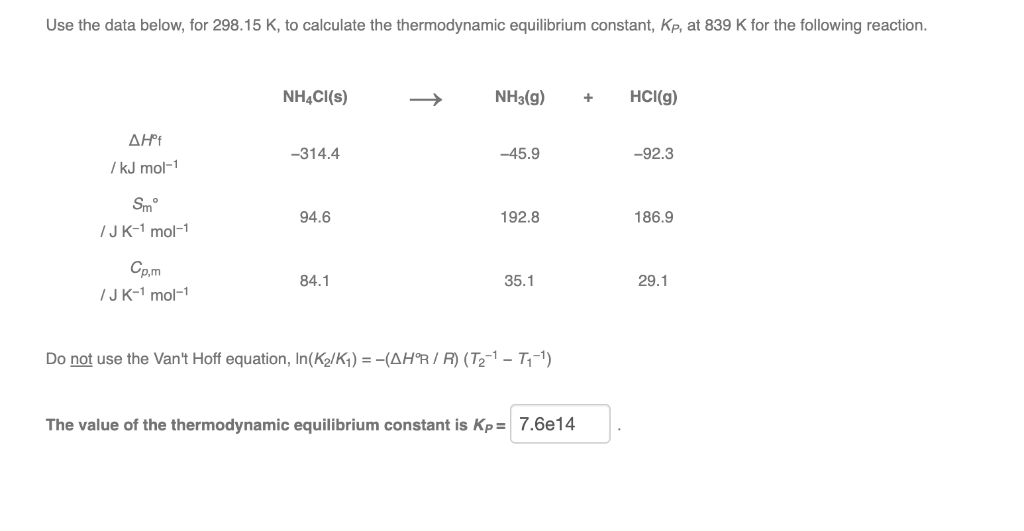Use the data below, for 298.15 K, to calculate the thermodynamic equilibrium constant, kp, at 839 K for the following reaction. NH4Cl(s) NH3(g) + HCl(g) ΔΗ 7 kJ mol-1 -314.4 -45.9 -92.3 Smº JK-1 mol-1 94.6 192.8 186.9 Cp,m 84.1 35.1 29.1 /JK-1 mol-1 Do not use the Van't Hoff equation, In(K /K1) = -(AHR/R) (T2-1 - 7,-1) The value of the thermodynamic equilibrium constant is Kp= 7.6e14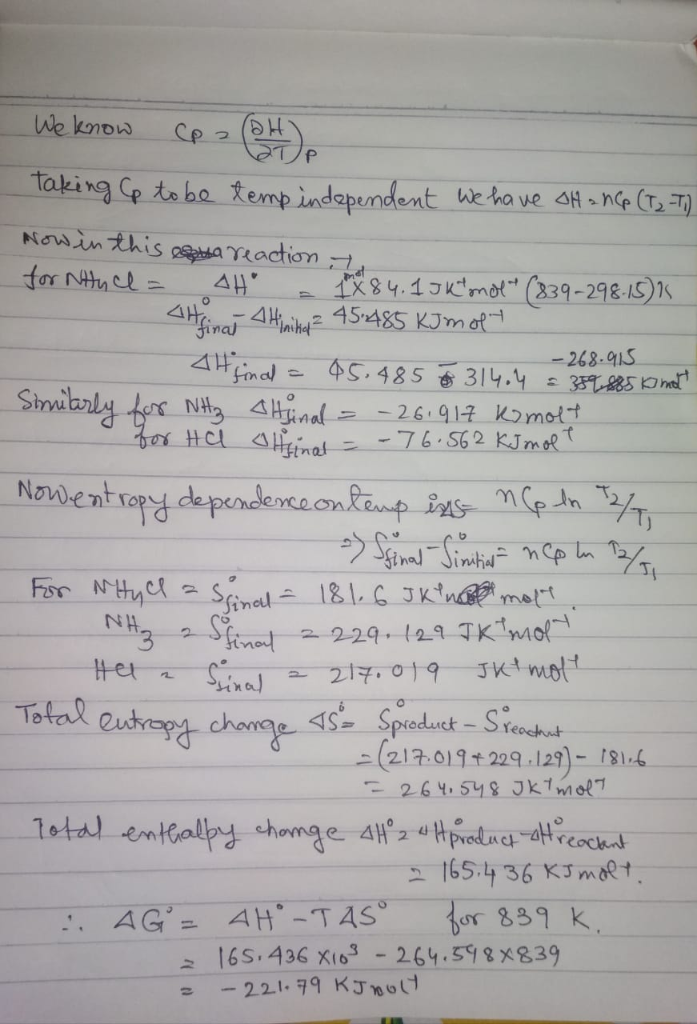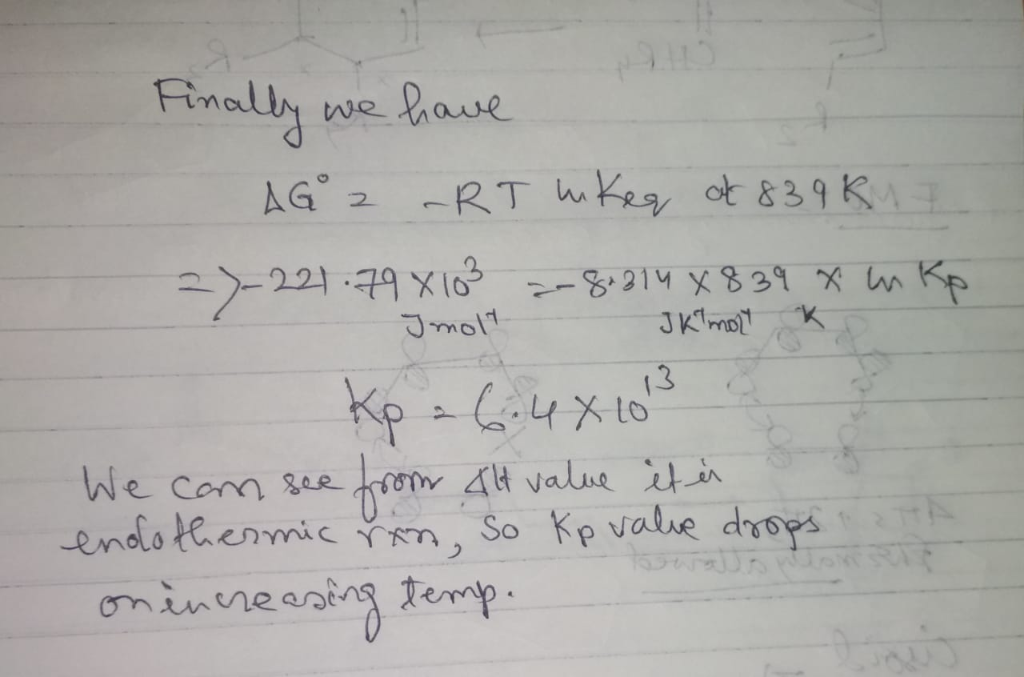Earn Coins

Coins can be redeemed for fabulous gifts.

Similar Homework Help Questions
• Use the data below, for 298.15 K, to calculate the thermodynamic equilibrium constant, kp, at 641...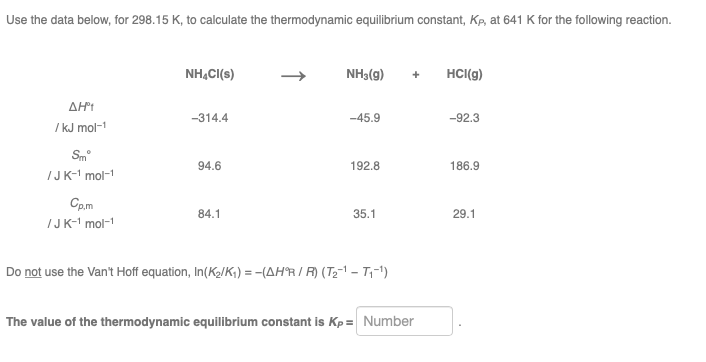Use the data below, for 298.15 K, to calculate the thermodynamic equilibrium constant, kp, at 641 K for the following reaction. NH4Cl(s) NH3(g) + HCl(g) ΔΗ /kJ mol-1 -314.4 -45.9 -92.3 Smº /JK-mol-1 94.6 192.8 186.9 Cp.m /JK-mol-1 84.1 35.1 29.1 Do not use the Van't Hoff equation, In(K/K) = -(AHR/R) (T2-1-T1-1) The value of the thermodynamic equilibrium constant is Kp = Number

• Using standard thermodynamic data (linked), calculate the equilibrium constant at 298.15 K for the following reaction....

Using standard thermodynamic data (linked), calculate the equilibrium constant at 298.15 K for the following reaction. HCl(g) + NH3(g)--->NH4Cl(s)      K =

• Use standard thermodynamic data (in the Chemistry References) to calculate AG at 298.15 K for the...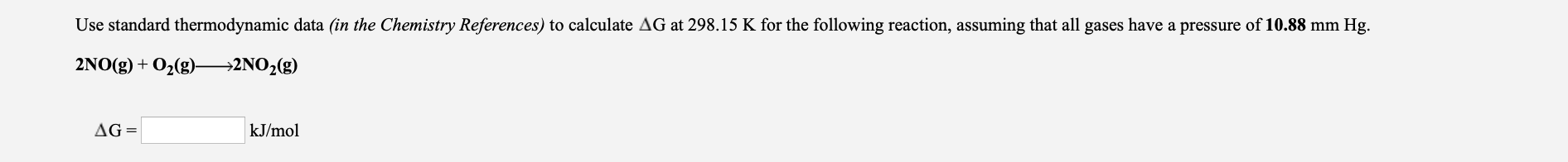Use standard thermodynamic data (in the Chemistry References) to calculate AG at 298.15 K for the following reaction, assuming that all gases have a pressure of 10.88 mm Hg. 2NO(g) + O2(g)—>2NO2(g) AG= kJ/mol Nitrogen AHºf (kJ/mol) AG°f (kJ/mol) sº (J/mol K) N2(g) 191.6 N(9) 472.7 455.6 153.3 NH3(9) -46.1 -16.5 192.5 NH3(aq) -80.0 -27.0 111.0 NH4+ (aq) -132.0 -79.0 113.0 NO(9) 90.3 86.6 210.8 NOCI(g) 51.7 66.1 261.8 NO2(9) 33.2 240.1 51.3 104.2 N2O(9) 82.1 219.9 N204(9) 9.2 97.9...

• Standard conditions 298.15 K (25 °C) and 1 bar. Thermodynamic Data Conversions Factors I cal 4.18...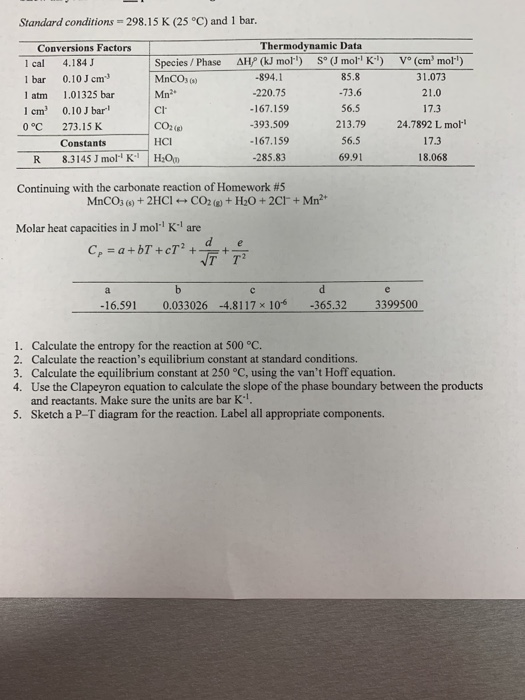Standard conditions 298.15 K (25 °C) and 1 bar. Thermodynamic Data Conversions Factors I cal 4.184 J 1 bar 0.10 J cm 1 atm 1.01325 bar 1 cm 0.10 J bar 0°C 273.15K Species/ Phase AHP J mol) MnCOs) Mn2 mo K) V° (cm mo) -894.1 -220.75 -167.159 -393.509 167.159 -285.83 85.8 73.6 56.5 213.79 56.5 69.91 31.073 21.0 17.3 24.7892 L mol 17.3 18.068 Cl CO2() Constants HCI R 8.3145 J mol! K-1 H:Op Continuing with the carbonate reaction...

• Standard conditions 298.15 K (25 °C) and 1 bar. Thermodynamic Data Conversions Factors I cal 4.184...Standard conditions 298.15 K (25 °C) and 1 bar. Thermodynamic Data Conversions Factors I cal 4.184 J 1 bar 0.10 J cm 1 atm 1.01325 bar 1 cm 0.10 J bar 0°C 273.15K Species/ Phase AHP J mol) MnCOs) Mn2 mo K) V° (cm mo) -894.1 -220.75 -167.159 -393.509 167.159 -285.83 85.8 73.6 56.5 213.79 56.5 69.91 31.073 21.0 17.3 24.7892 L mol 17.3 18.068 Cl CO2() Constants HCI R 8.3145 J mol! K-1 H:Op Continuing with the carbonate reaction...

• Use standard thermodynamic data (in the Chemistry References) to calculate G at 298.15 K for the...

Use standard thermodynamic data (in the Chemistry References) to calculate G at 298.15 K for the following reaction, assuming that all gases have a pressure of 14.96 mm Hg. 2N2(g) + O2(g)2N2O(g) G =_____ kJ/mol

• Given the information below, calculate ∆?∘?,298.15? and ∆?∘?,400? for the following reaction: ??(?) + 2???(?) →...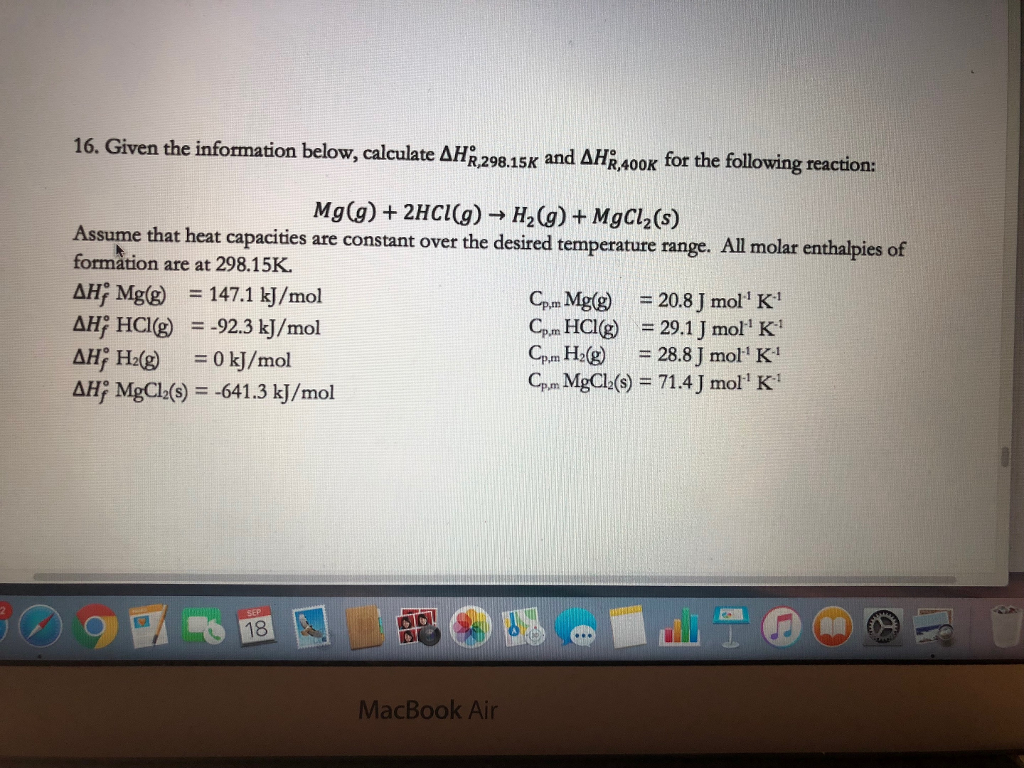Given the information below, calculate ∆?∘?,298.15? and ∆?∘?,400? for the following reaction: ??(?) + 2???(?) → ?2(?) + ????2(?) Assume that heat capacities are constant over the desired temperature range. All molar enthalpies of formation are at 298.15K. ∆?∘ Mg(g) ? = 147.1 kJ/mol ∆?∘ HCl(g) ? = -92.3 kJ/mol ∆?∘ H2(g) ? = 0 kJ/mol ∆?∘ MgCl2(s) = -641.3 kJ/mol Cp,m Mg(g) = 20.8 J mol-1 K-1 Cp,m HCl(g) = 29.1 J mol-1 K-1 Cp,m H2(g) = 28.8 J...

• 7 Consider the following reaction at 298 K: NH3(g) +HCI(g)NH4CI(s) Using the thermochemical data at 298 K given below (a) Determine the AH for the reaction. (2 marks) (b) Determine the AG for the...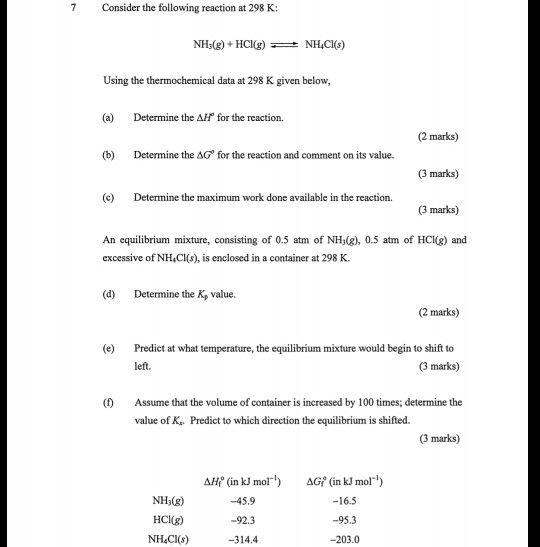7 Consider the following reaction at 298 K: NH3(g) +HCI(g)NH4CI(s) Using the thermochemical data at 298 K given below (a) Determine the AH for the reaction. (2 marks) (b) Determine the AG for the reaction and comment on its value. (3 marks) (c) Determine the maximum work done available in the reaction. (3 marks) An equilibrium mixture, consisting of 0.5 atm of NHy(), 0.5 atm of HCKg) and excessive of NH Cl(s), is enclosed in a container at 298 K...

• Calculate the equilibrium constant from the standard free energy change. Using standard thermodynamic data (linked), calculate...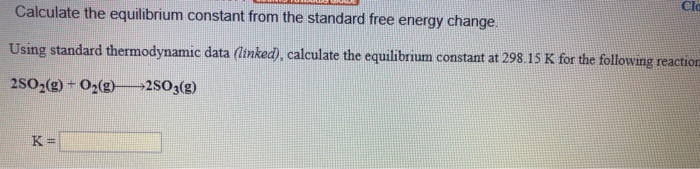Calculate the equilibrium constant from the standard free energy change. Using standard thermodynamic data (linked), calculate the equilibrium constant at 298.15 K for the following reaction 2502(g) + O2(0) 2503(9) AH°F (kJ/mol) AGⓇ, (kJ/mol sº (/mol K) 205.1 Oxygen 02(0) (9) O3(9) 249.2 231.7 161.1 142.7 163.2 238.9 AH°: (kJ/mol Agºr (kJ/mol) 5° (J/mol K) 31.8 0.3 0.3 278.8 3 3.0 167.8 33.0 - 15.0 Sulfur S(s, rhombic) S(s, monodinic) S(g) Sz"(aq) Sg(9) SzCl2(g) SF6(9) H2S(9) soz(9) SO3(0) 1 0....

• Use the data from this table of thermodynamic properties to calculate the values of ASixn for...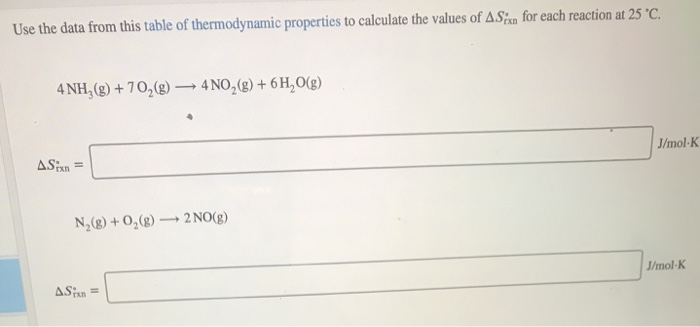Use the data from this table of thermodynamic properties to calculate the values of ASixn for each reaction at 25 °C. 4NH,(g) + 70,(9) — 4NO2(g) + 6H2O(g) AS:n = J/mol K N,() + 0,(g) 2 NO(g) J/mol K Asian = MISU415) -1065.25 N2(9) N2H4(0) 50.6 N20(9) 81.6 N204(9) 11.1 N205(9) 13.3 NH3(9) -45.9 NH3(aq) -80.29 NH,+(aq) -133.3 NH4CI(S) -314.4 NH4NO3(s) -365.6 (NH2)2CO(s) -333.1 Na(s) Na+(aq) -240.3 Na2CO3(S) -1130.7 NaCl(s) -411.2 NaF(s) -576.6 NaBr(s) -361.1 Nal(s) -287.8 NaNO3(s) -467.9 NaOH(s)...